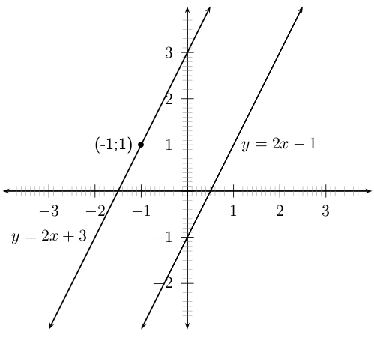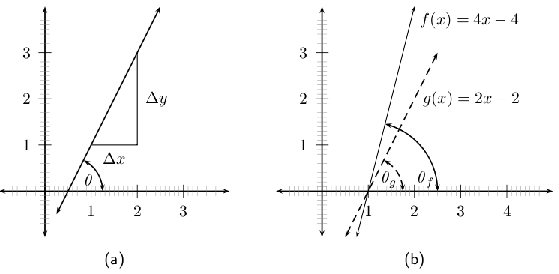# 4.2 Co-ordinate geometry

 Page 1 / 1

## Equation of a line between two points

There are many different methods of specifying the requirements for determining the equation of a straight line. One option is to find the equation of a straight line, when two points are given.

Assume that the two points are $\left({x}_{1};{y}_{1}\right)$ and $\left({x}_{2};{y}_{2}\right)$ , and we know that the general form of the equation for a straight line is:

$y=mx+c$

So, to determine the equation of the line passing through our two points, we need to determine values for $m$ (the gradient of the line) and $c$ (the $y$ -intercept of the line). The resulting equation is

$y-{y}_{1}=m\left(x-{x}_{1}\right)$

where $\left({x}_{1};{y}_{1}\right)$ are the co-ordinates of either given point.

## Finding the second equation for a straight line

This is an example of a set of simultaneous equations, because we can write:

$\begin{array}{ccc}\hfill {y}_{1}& =& m{x}_{1}+c\hfill \\ \hfill {y}_{2}& =& m{x}_{2}+c\hfill \end{array}$

We now have two equations, with two unknowns, $m$ and $c$ .

$\begin{array}{ccc}\hfill {\mathrm{y}}_{2}-{\mathrm{y}}_{1}& =& m{x}_{2}-m{x}_{1}\hfill \\ \hfill \therefore \phantom{\rule{1.em}{0ex}}m& =& \frac{{y}_{2}-{y}_{1}}{{x}_{2}-{x}_{1}}\hfill \\ \hfill {\mathrm{y}}_{1}& =& m{x}_{1}+c\hfill \\ \hfill c& =& {y}_{1}-m{x}_{1}\hfill \end{array}$

Now, to make things a bit easier to remember, substitute [link] into [link] :

$\begin{array}{ccc}\hfill y& =& mx+c\hfill \\ & =& mx+\left({y}_{1}-m{x}_{1}\right)\hfill \\ \hfill \mathrm{y}-{\mathrm{y}}_{1}& =& m\left(x-{x}_{1}\right)\hfill \end{array}$

If you are asked to calculate the equation of a line passing through two points, use:

$m=\frac{{y}_{2}-{y}_{1}}{{x}_{2}-{x}_{1}}$

to calculate $m$ and then use:

$y-{y}_{1}=m\left(x-{x}_{1}\right)$

to determine the equation.

For example, the equation of the straight line passing through $\left(-1;1\right)$ and $\left(2;2\right)$ is given by first calculating $m$

$\begin{array}{ccc}\hfill m& =& \frac{{y}_{2}-{y}_{1}}{{x}_{2}-{x}_{1}}\hfill \\ & =& \frac{2-1}{2-\left(-1\right)}\hfill \\ & =& \frac{1}{3}\hfill \end{array}$

and then substituting this value into

$y-{y}_{1}=m\left(x-{x}_{1}\right)$

to obtain

$\begin{array}{ccc}\hfill y-{y}_{1}& =& \frac{1}{3}\left(x-{x}_{1}\right).\hfill \end{array}$

Then substitute $\left(-1;1\right)$ to obtain

$\begin{array}{ccc}\hfill y-\left(1\right)& =& \frac{1}{3}\left(x-\left(-1\right)\right)\hfill \\ \hfill y-1& =& \frac{1}{3}x+\frac{1}{3}\hfill \\ \hfill y& =& \frac{1}{3}x+\frac{1}{3}+1\hfill \\ \hfill y& =& \frac{1}{3}x+\frac{4}{3}\hfill \end{array}$

So, $y=\frac{1}{3}x+\frac{4}{3}$ passes through $\left(-1;1\right)$ and $\left(2;2\right)$ .

Find the equation of the straight line passing through $\left(-3;2\right)$ and $\left(5;8\right)$ .

1. $\begin{array}{ccc}\hfill \left({x}_{1};{y}_{1}\right)& =& \left(-3;2\right)\hfill \\ \hfill \left({x}_{2};{y}_{2}\right)& =& \left(5;8\right)\hfill \end{array}$
2. $\begin{array}{ccc}\hfill m& =& \frac{{y}_{2}-{y}_{1}}{{x}_{2}-{x}_{1}}\hfill \\ & =& \frac{8-2}{5-\left(-3\right)}\hfill \\ & =& \frac{6}{5+3}\hfill \\ & =& \frac{6}{8}\hfill \\ & =& \frac{3}{4}\hfill \end{array}$
3. $\begin{array}{ccc}\hfill y-{y}_{1}& =& m\left(x-{x}_{1}\right)\hfill \\ \hfill y-\left(2\right)& =& \frac{3}{4}\left(x-\left(-3\right)\right)\hfill \\ \hfill y& =& \frac{3}{4}\left(x+3\right)+2\hfill \\ & =& \frac{3}{4}x+\frac{3}{4}·3+2\hfill \\ & =& \frac{3}{4}x+\frac{9}{4}+\frac{8}{4}\hfill \\ & =& \frac{3}{4}x+\frac{17}{4}\hfill \end{array}$
4. The equation of the straight line that passes through $\left(-3;2\right)$ and $\left(5;8\right)$ is $y=\frac{3}{4}x+\frac{17}{4}$ .

## Equation of a line through one point and parallel or perpendicular to another line

Another method of determining the equation of a straight-line is to be given one point, $\left({x}_{1};{y}_{1}\right)$ , and to be told that the line is parallel or perpendicular to another line. If the equation of the unknown line is $y=mx+c$ and the equation of the second line is $y={m}_{0}x+{c}_{0}$ , then we know the following:

$\begin{array}{ccc}\hfill \mathrm{If the lines are parallel, then}\phantom{\rule{1.em}{0ex}}\mathrm{m}& =& {m}_{0}\hfill \\ \hfill \mathrm{If the lines are perpendicular, then}\phantom{\rule{1.em}{0ex}}\mathrm{m}×{\mathrm{m}}_{0}& =& -1\hfill \end{array}$

Once we have determined a value for $m$ , we can then use the given point together with:

$y-{y}_{1}=m\left(x-{x}_{1}\right)$

to determine the equation of the line.

For example, find the equation of the line that is parallel to $y=2x-1$ and that passes through $\left(-1;1\right)$ .

First we determine $m$ , the slope of the line we are trying to find. Since the line we are looking for is parallel to $y=2x-1$ ,

$m=2$

The equation is found by substituting $m$ and $\left(-1;1\right)$ into:

$\begin{array}{ccc}\hfill y-{y}_{1}& =& m\left(x-{x}_{1}\right)\hfill \\ \hfill y-1& =& 2\left(x-\left(-1\right)\hfill \\ \hfill y-1& =& 2\left(x+1\right)\hfill \\ \hfill y-1& =& 2x+2\hfill \\ \hfill y& =& 2x+2+1\hfill \\ \hfill y& =& 2x+3\hfill \end{array}$The equation of the line passing through ( - 1 ; 1 ) and parallel to y = 2 x - 1 is y = 2 x + 3 . It can be seen that the lines are parallel to each other. You can test this by using your ruler and measuring the perpendicular distance between the lines at different points.

## Inclination of a line(a) A line makes an angle θ with the x -axis. (b) The angle is dependent on the gradient. If the gradient of f is m f and the gradient of g is m g then m f > m g and θ f > θ g .

In [link] (a), we see that the line makes an angle $\theta$ with the $x$ -axis. This angle is known as the inclination of the line and it is sometimes interesting to know what the value of $\theta$ is.

Firstly, we note that if the gradient changes, then the value of $\theta$ changes ( [link] (b)), so we suspect that the inclination of a line is related to the gradient. We know that the gradient is a ratio of a change in the $y$ -direction to a change in the $x$ -direction.

$m=\frac{\Delta y}{\Delta x}$

But, in [link] (a) we see that

$\begin{array}{ccc}\hfill tan\theta & =& \frac{\Delta y}{\Delta x}\hfill \\ \hfill \therefore m& =& tan\theta \hfill \end{array}$

For example, to find the inclination of the line $y=x$ , we know $m=1$

$\begin{array}{ccc}\hfill \therefore tan\theta & =& 1\hfill \\ \hfill \therefore \theta & =& {45}^{\circ }\hfill \end{array}$

## Co-ordinate geometry

1. Find the equations of the following lines
1. through points $\left(-1;3\right)$ and $\left(1;4\right)$
2. through points $\left(7;-3\right)$ and $\left(0;4\right)$
3. parallel to $y=\frac{1}{2}x+3$ passing through $\left(-1;3\right)$
4. perpendicular to $y=-\frac{1}{2}x+3$ passing through $\left(-1;2\right)$
5. perpendicular to $2y+x=6$ passing through the origin
2. Find the inclination of the following lines
1. $y=2x-3$
2. $y=\frac{1}{3}x-7$
3. $4y=3x+8$
4. $y=-\frac{2}{3}x+3$ (Hint: if $m$ is negative $\theta$ must be in the second quadrant)
5. $3y+x-3=0$
3. Show that the line $y=k$ for any constant $k$ is parallel to the x-axis. (Hint: Show that the inclination of this line is ${0}^{\circ }$ .)
4. Show that the line $x=k$ for any constant $k$ is parallel to the y-axis. (Hint: Show that the inclination of this line is ${90}^{\circ }$ .)

what is variations in raman spectra for nanomaterials
I only see partial conversation and what's the question here!
what about nanotechnology for water purification
please someone correct me if I'm wrong but I think one can use nanoparticles, specially silver nanoparticles for water treatment.
Damian
yes that's correct
Professor
I think
Professor
what is the stm
is there industrial application of fullrenes. What is the method to prepare fullrene on large scale.?
Rafiq
industrial application...? mmm I think on the medical side as drug carrier, but you should go deeper on your research, I may be wrong
Damian
How we are making nano material?
what is a peer
What is meant by 'nano scale'?
What is STMs full form?
LITNING
scanning tunneling microscope
Sahil
how nano science is used for hydrophobicity
Santosh
Do u think that Graphene and Fullrene fiber can be used to make Air Plane body structure the lightest and strongest. Rafiq
Rafiq
what is differents between GO and RGO?
Mahi
what is simplest way to understand the applications of nano robots used to detect the cancer affected cell of human body.? How this robot is carried to required site of body cell.? what will be the carrier material and how can be detected that correct delivery of drug is done Rafiq
Rafiq
what is Nano technology ?
write examples of Nano molecule?
Bob
The nanotechnology is as new science, to scale nanometric
brayan
nanotechnology is the study, desing, synthesis, manipulation and application of materials and functional systems through control of matter at nanoscale
Damian
Is there any normative that regulates the use of silver nanoparticles?
what king of growth are you checking .?
Renato
What fields keep nano created devices from performing or assimulating ? Magnetic fields ? Are do they assimilate ?
why we need to study biomolecules, molecular biology in nanotechnology?
?
Kyle
yes I'm doing my masters in nanotechnology, we are being studying all these domains as well..
why?
what school?
Kyle
biomolecules are e building blocks of every organics and inorganic materials.
Joe
anyone know any internet site where one can find nanotechnology papers?
research.net
kanaga
sciencedirect big data base
Ernesto
Introduction about quantum dots in nanotechnology
what does nano mean?
nano basically means 10^(-9). nanometer is a unit to measure length.
Bharti
do you think it's worthwhile in the long term to study the effects and possibilities of nanotechnology on viral treatment?
absolutely yes
Daniel
how did you get the value of 2000N.What calculations are needed to arrive at it
Privacy Information Security Software Version 1.1a
Good
Other chapter Q/A we can askByByByByByByByBy